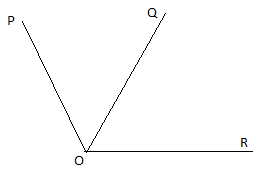QUESTION

# How many angles are shown in the figure? Name them.If we consider two rays OP and OR, they both make an angle at point O, which called as $\angle POR$
Now, If we consider two rays OR and OQ, they both make an angle at point O, which called as $\angle ROQ$
Similarly, If we consider two rays OP and OQ, they both make an angle at point O, which called as $\angle POQ$
Hence, in the given figure there are three angles that are named as $\angle POR,\angle ROQ{\text{ and }}\angle POQ.$